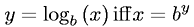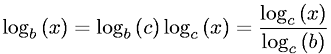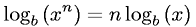Algebra > LogarithmsShowing results 1 to 7 of 7, on page 1 of 1
 DESCRIPTION EQUATION Definition of a LogarithmDifference of Logarithms PropertyLogarithm Base ConversionLogarithm of an ExponentialLogarithm of OneLogarithmic Identity PropertySum of Logarithms Property